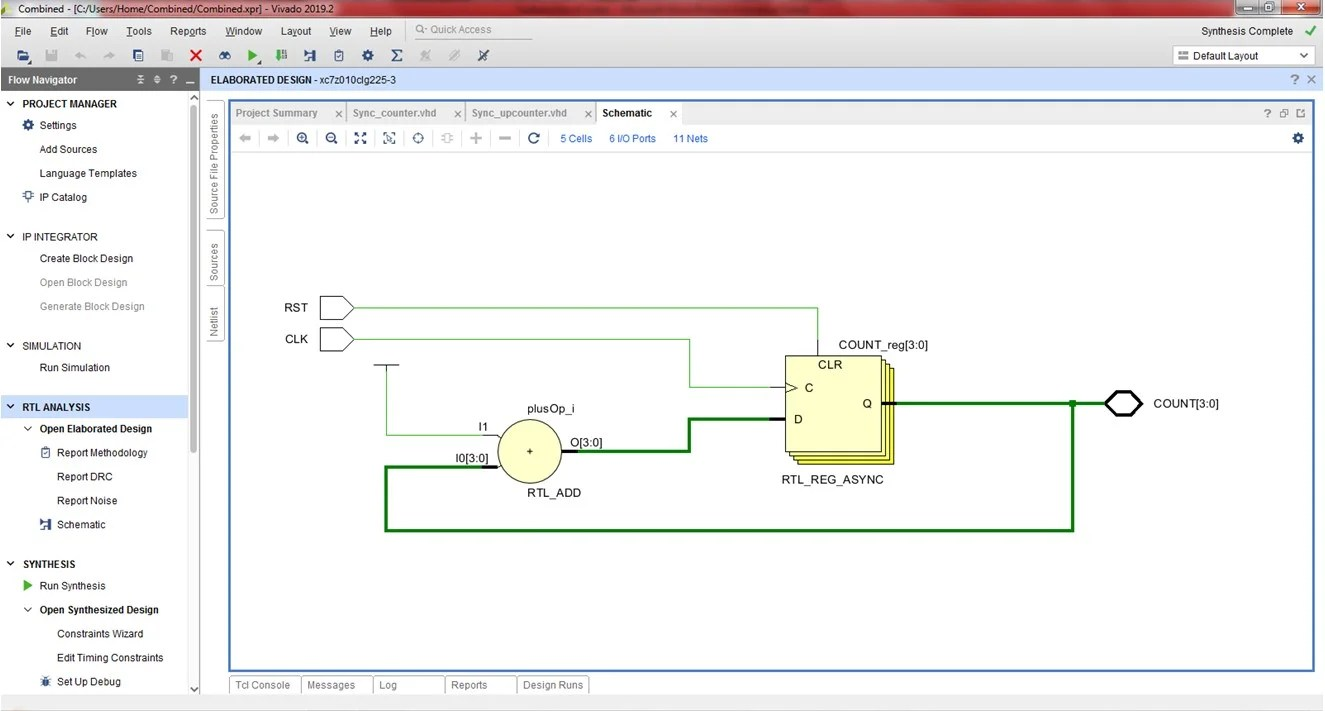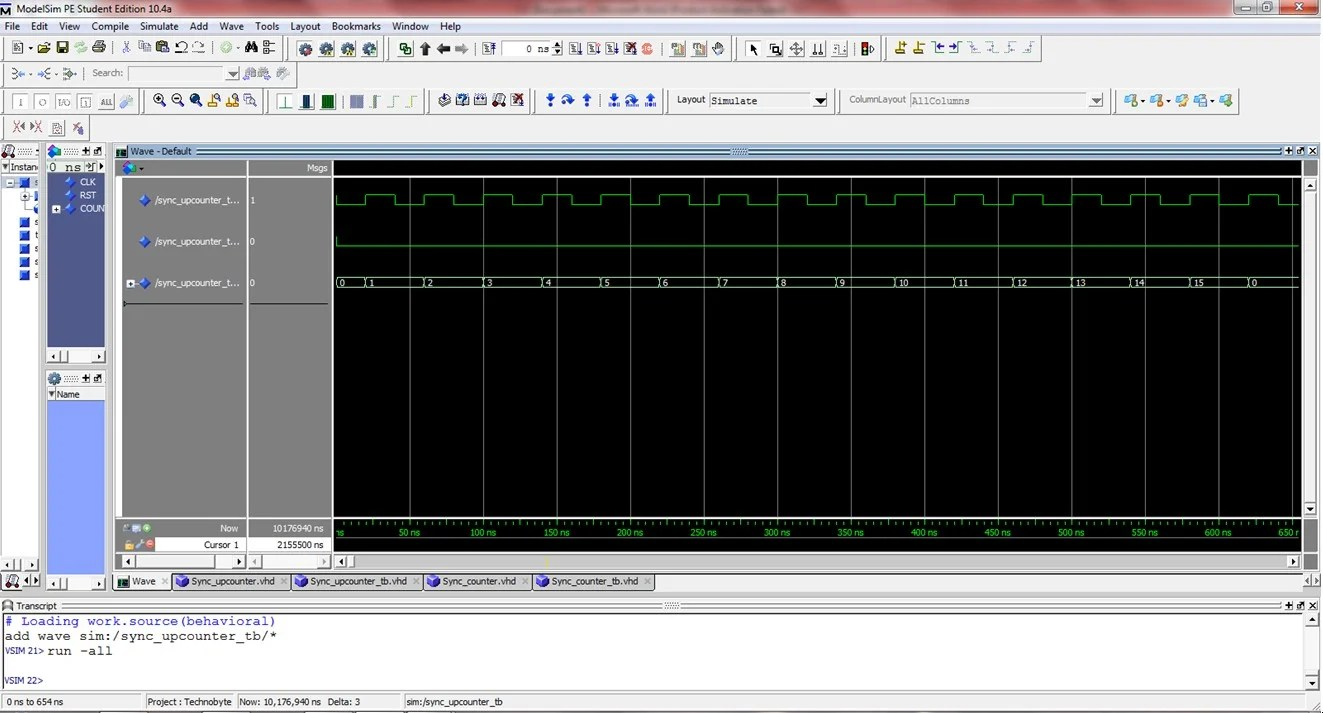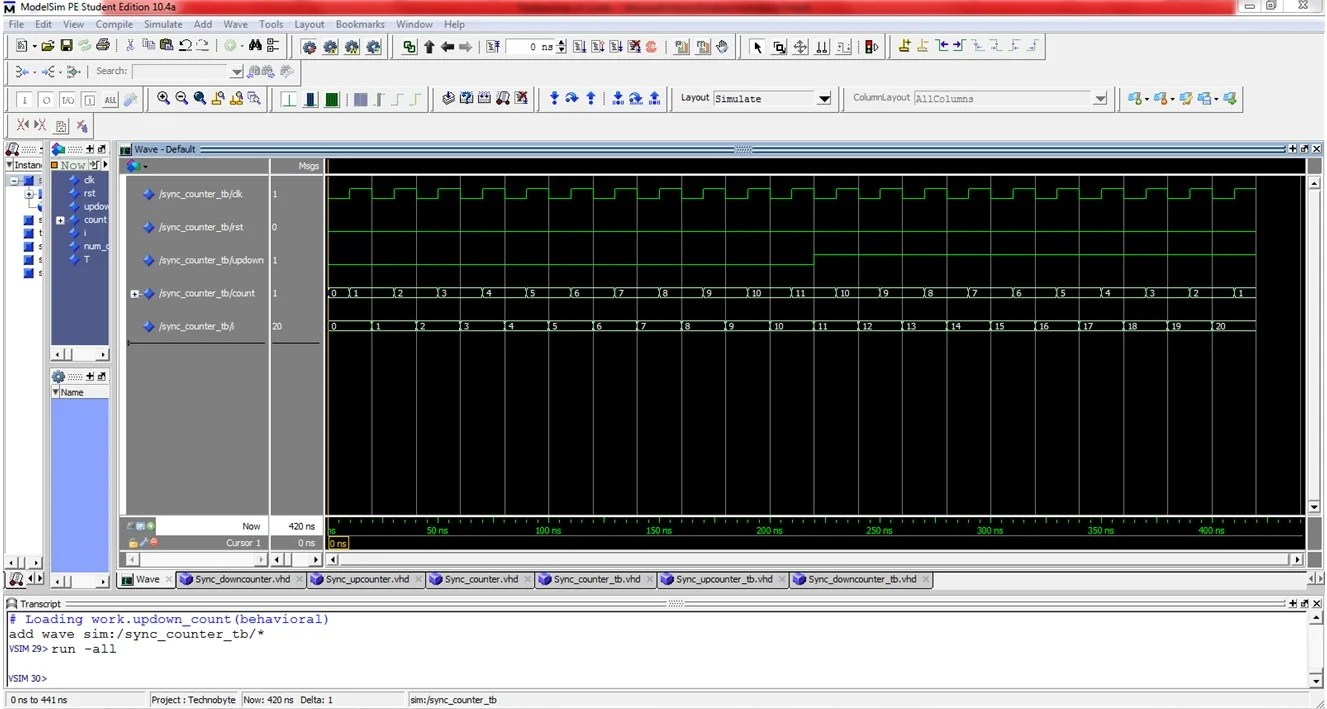View Course Path

# VHDL code for synchronous counters: Up, down, up-down (Behavioral)

Let’s take a look at implementing the VHDL code for synchronous counters using behavioral architecture. Counters are sequential circuits that employ a cascade of flip-flops that are used to count something. We will write the VHDL code for all the three types of synchronous counters: up, down, and up-down. First, we will take a look at their logic circuits. Then we will write the VHDL code, then test the code using testbenches. Finally, we will synthesize the RTL schematic and the simulation waveforms.

Contents

## Up-counter

### Explanation of the VHDL code for synchronous up-counter using behavioral modeling method. How does the code work?

Synchronous means to be driven by the same clock. The flip-flops in the synchronous counters are all driven by a single clock input. You can see the logic circuit of the 4-bit synchronous up-counter above. It has two inputs of STD_LOGIC, Clock and Reset. And four outputs since its a 4-bit counter.

Since these 4-bits are similar, we will declare them using the `STD_LOGIC_VECTOR` data type. The direction is in/out because the previous count will be considered to increase the subsequent count. And in that case, it is also an input.

Since we are using behavioral architecture, we will define the behavior of the circuit using if-elsif statements. The `process` statement has the inputs in its sensitivity list.

```library IEEE;
use IEEE.STD_LOGIC_1164.ALL;
use IEEE.STD_LOGIC_ARITH.ALL;
use IEEE.STD_LOGIC_UNSIGNED.ALL;

entity SOURCE is
Port ( CLK,RST : in  STD_LOGIC;
COUNT : inout  STD_LOGIC_VECTOR (3 downto 0));
end SOURCE;

architecture Behavioral of SOURCE is

begin
process (CLK,RST)
begin
```

The main program is very simple and straightforward. When the reset signal is active, the count will be reset to “0000”. When it’s not, and the clock is on a rising edge, the current value of the counter will be obtained by incrementing the previous value by one.

```if (RST = '1')then
COUNT <= "0000";
elsif(rising_edge(CLK))then
COUNT <= COUNT+1;
```

Finally, the closing statements:

```end if;
end process;
end Behavioral;
```

## Full VHDL code for synchronous up-counter using the behavioral method

```library IEEE;
use IEEE.STD_LOGIC_1164.ALL;
use IEEE.STD_LOGIC_ARITH.ALL;
use IEEE.STD_LOGIC_UNSIGNED.ALL;

entity SOURCE is
Port ( CLK,RST : in  STD_LOGIC;
COUNT : inout  STD_LOGIC_VECTOR (3 downto 0));
end SOURCE;

architecture Behavioral of SOURCE is

begin
process (CLK,RST)
begin

if (RST = '1')then
COUNT <= "0000";
elsif(rising_edge(CLK))then
COUNT <= COUNT+1;

end if;
end process;
end Behavioral;
```

### Testbench

We will use the infinite testbench type of testbench as we had discussed in our guide on writing a VHDL testbench.

```library IEEE;
use IEEE.STD_LOGIC_1164.ALL;
use IEEE.STD_LOGIC_ARITH.ALL;
use IEEE.STD_LOGIC_UNSIGNED.ALL;

entity sync_upcounter_tb is
end entity;

architecture tb of sync_upcounter_tb is
component SOURCE is
Port ( CLK,RST : in STD_LOGIC;
COUNT : inout STD_LOGIC_VECTOR (3 downto 0));
end component;

signal CLK,RST : STD_LOGIC := '1';
signal COUNT : STD_LOGIC_VECTOR(3 downto 0);

begin

uut: SOURCE port map(
CLK => CLK,
RST => RST,
COUNT => COUNT);

clock: process
begin

RST <= '0';

CLK <= '0';
wait for 20 ns;
CLK <= '1';
wait for 20 ns;

end process;
end tb;```

### RTL Schematic### Simulation WaveformsAfter coding the up-counter, we will implement the VHDL code for synchronous down counter using behavioral architecture. First, will understand its behavior. And then we will understand the syntax. For the full code, scroll down.

## Down-counter

The 4-bit synchronous down counter counts in decrements of 1. The maximum count that it can countdown from is 16 (i.e. 0-15).

The 4-bit down counter is very much similar to the circuit of the 4-bit up-counter. The only difference is that in the down counter, you have to attach the nQ outputs of the D flip-flop to the display. Instead of the Q (uncomplemented) outputs as we did in up-counter.

### Explanation of the VHDL code for synchronous down counter using the behavioral method. How does the code work?

We are going to try a little something different over here. Technically, we can recycle the code from above and replace COUNT=>COUNT+1 by COUNT=>COUNT-1. But that wouldn’t allow us to learn. Here, we will code an almost similar program to the one above. However, the only difference is that here, we will be using a variable signal. That’s a thing in VHDL. Check it out below.

The variable signal will be a placeholder for the circuit to remember its previous value. Once its use is done, we will assign the values to the proper ports. The variable signal is declared after the architecture statement. This is kind of like the global declaration concept. Kind of. Declaring it before initiating the process gives us the chance to use it in other processes. If we wish to do so.

The entity architecture pair is just the same as it was for the 4-bit up-counter except for a minor change. Since we are using a variable signal, we no longer need to declare the ‘COUNT’ output as ‘inout’. The rest stays the same. The ‘temp’ variable signal is a placeholder for the output count. So it will be of four bits too.

```library IEEE;
use IEEE.STD_LOGIC_1164.ALL;
use IEEE.STD_LOGIC_ARITH.ALL;
use IEEE.STD_LOGIC_UNSIGNED.ALL;
entity down_count is
Port ( clk,rst : in  STD_LOGIC;
count : out  STD_LOGIC_VECTOR (3 downto 0));
end down_count;

architecture Behavioral of up_count is

signal temp:std_logic_vector(3 downto 0);
begin
process(clk,rst)
begin
```

Another distinct yet obvious detail that you need to keep in mind is that since this is a down-counter, its reset state would be at “1111”.

```if(rst='1')then
temp<="1111";
```

The rest of the code is as follows. We decrement the count for every clock cycle. At the end of the process, we assign the `temp` variable signal to the `count` output port.

```elsif(rising_edge(clk))then
temp<=temp-1;
end if;
end process;
count<=temp;
end Behavioral;
```

### Full VHDL code for synchronous down counter using behavioral modeling method

```library IEEE;
use IEEE.STD_LOGIC_1164.ALL;
use IEEE.STD_LOGIC_ARITH.ALL;
use IEEE.STD_LOGIC_UNSIGNED.ALL;
entity down_count is
Port ( clk,rst : in  STD_LOGIC;
count : out  STD_LOGIC_VECTOR (3 downto 0));
end down_count;

architecture Behavioral of down_count is

signal temp:std_logic_vector(3 downto 0);
begin
process(clk,rst)
begin

if(rst='1')then
temp<="1111";
elsif(rising_edge(clk))then
temp<=temp-1;
end if;
end process;

count<=temp;
end Behavioral;
```

### Testbench

We will again use the infinite testbench type of testbench here.

```library IEEE;
use IEEE.STD_LOGIC_1164.ALL;
use IEEE.STD_LOGIC_ARITH.ALL;
use IEEE.STD_LOGIC_UNSIGNED.ALL;

entity sync_downcounter_tb is
end entity;

architecture tb of sync_downcounter_tb is
component down_count is
Port ( clk,rst : in STD_LOGIC;
count : out STD_LOGIC_VECTOR (3 downto 0));
end component;

signal clk, rst : STD_LOGIC := '1';
signal count : STD_LOGIC_VECTOR(3 downto 0);

begin

uut: down_count port map(
clk => clk,
rst => rst,
count => count);

clock: process
begin

rst <= '0';

clk <= '0';
wait for 20 ns;
clk <= '1';
wait for 20 ns;

end process;
end tb;```

### RTL Schematic### Simulation WaveformFinally, we will combine the up-counter and the down counter. Accordingly, we will write the VHDL code for synchronous up-down counter using behavioral architecture. We will understand the syntax. For the full code, scroll down.

## Up-down counter

### Explanation of the VHDL code for synchronous up-down counter using the behavioral modeling method. How does the code work?

If you have diligently followed our course on digital electronics and digital logic design, and this course on VHDL coding, then you should be able to figure out a circuit for this right away. Let’s play. Think about it. We already have two circuits. One counts up. Another counts down. How can we combine them to get something that does both? Hint: how to choose between two outputs?

A multiplexer is the right answer! If we attach a four 2:1 multiplexers for every flip-flops two outputs, we can control which output goes to the display using one select line. Check out the diagram below for a 3-bit synchronous up-down counter to get an idea. You should be able to design a 4-bit equivalent once you’ve seen this.

### Full VHDL code for synchronous up-down counter using the behavioral method

```library IEEE;
use IEEE.STD_LOGIC_1164.ALL;
use IEEE.STD_LOGIC_ARITH.ALL;
use IEEE.STD_LOGIC_UNSIGNED.ALL;

entity updown_count is
Port ( clk,rst,updown : in  STD_LOGIC;
count : out  STD_LOGIC_VECTOR (3 downto 0));
end updown_count;

architecture Behavioral of updown_count is

signal temp:std_logic_vector(3 downto 0):="0000";

begin
process(clk,rst)
begin

if(rst='1')then
temp<="0000";
elsif(rising_edge(clk))then
if(updown='0')then
temp<=temp+1;
else
temp<=temp-1;
end if;
end if;
end process;

count<=temp;
end Behavioral;```

### Testbench

For the up-down counter, we will use the finite testbench type of testbench.

```library IEEE;
use IEEE.STD_LOGIC_1164.ALL;
use IEEE.STD_LOGIC_ARITH.ALL;
use IEEE.STD_LOGIC_UNSIGNED.ALL;

entity sync_counter_tb is
end entity;

architecture tb of sync_counter_tb is
component updown_count is
Port ( clk,rst,updown : in STD_LOGIC;
count : out STD_LOGIC_VECTOR (3 downto 0));
end component;

signal clk, rst, updown : STD_LOGIC := '0';
signal count : STD_LOGIC_VECTOR (3 downto 0);
constant num_of_clocks : integer := 20;
signal i : integer := 0;
constant T : time := 20 ns;

begin

uut: updown_count port map(
clk => clk,
rst => rst,
updown => updown,
count => count);

process
begin
rst <= '0';
clk <= '0';
wait for T/2;
clk <= '1';
wait for T/2;

if (i = num_of_clocks) then
wait;
else
i <= i + 1;
end if;

if (i < 10) then
updown <= '0';
else
updown <= '1';
end if;

end process;
end tb;```

### RTL Schematic### Simulation WaveformEdit: Post updated with the testbench, RTL Schematic, and Simulation Waveform by Deepak Joshi.

This site uses Akismet to reduce spam. Learn how your comment data is processed.

Top